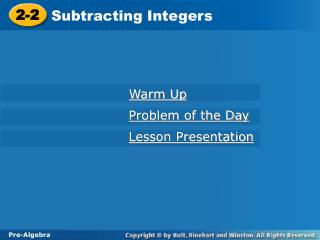DownloadDownload Presentation2-2

# 2-2

Télécharger la présentation## 2-2

- - - - - - - - - - - - - - - - - - - - - - - - - - - E N D - - - - - - - - - - - - - - - - - - - - - - - - - - -
##### Presentation Transcript

1. 2-2 Subtracting Integers Pre-Algebra Warm Up Problem of the Day Lesson Presentation

2. 2-2 Subtracting Integers Pre-Algebra Warm Up Add. 1. –7 + 2 4. –6 + (–28) 2. –12 + (–9) 5. 104 + (–87) 3. 32 +(–19) 6. –18 + (–24) –5 –34 –21 17 13 –42

3. +5 +2 +8 –5 Problem of the Day Copy and complete the magic square. The magic sum is 0.

4. Learn to subtract integers.

5. Subtracting a smaller number from a larger number is the same as finding how far apart the two numbers are on a number line. Subtracting an integer is the same as adding its opposite.

6. Additional Example 1: Subtracting Integers Subtract. A. –7 – 4 –7 – 4 = –7 + (–4) Add the opposite of 4. = –11 Same sign; use the sign of the integers. B. 8 – (–5) 8 – (–5) = 8 + 5 Add the opposite of –5. = 13 Same sign; use the sign of the integers. C.–6 – (–3) –6 – (–3) = –6 + 3 Add the opposite of –3. = –3 6 > 3; use the sign of 6.

7. Try This: Example 1 Subtract. A. 3 – (–6) 3 – (–6) = 3 + 6 Add the opposite of –6. Same signs; use the sign of the integers. = 9 B. –4 – 1 –4 – 1 = –4 + (–1) Add the opposite of 1. = –5 Same sign; use the sign of the integers. C.–7 – (–8) –7 – (–8) = –7 + 8 Add the opposite of –8. = 1 8 > 7; use the sign of 8.

8. Additional Example 2A: Evaluating Expressions with Integers Evaluate the expression for the given value of the variable. A. 8 –jfor j = –6 8 –j 8 – (–6) Substitute –6 for j. = 8 + 6 Add the opposite of –6. = 14 Same sign; use the sign of the integers.

9. Additional Example 2B: Evaluating Expressions with Integers Evaluate the expression for the given value of the variable. B. –9 – y for y = –4 –9 – y –9 – (–4) Substitute –4 for y. = –9 + 4 Add the opposite of –4. = –5 9 > 4; use the sign of 9.

10. Additional Example 2C: Evaluating Expressions with Integers Evaluate each expression for the given value of the variable. C.n–6for n = –2 n–6 –2– 6 Substitute –2 for n. = –2 + (–6) Add the opposite of 6. = –8 Same sign; use the sign of the integers.

11. Try This: Example 2A Evaluate the expression for the given value of the variable. A. 11 –mfor m = –3 11 –m 11 – (–3) Substitute –3 for m. = 11 + 3 Add the opposite of –3. = 14 Same sign; use the sign of the integers.

12. Try This: Example 2B Evaluate the expression for the given value of the variable. B. –5 – r for r = –2 –5 – r –5 – (–2) Substitute –2 for r. = –5 + 2 Add the opposite of –2. = –3 5 > 2; use the sign of 5.

13. Try This: Example 2C Evaluate each expression for the given value of the variable. C.a–7for a = –9 a– 7 –9– 7 Substitute –9 for a. = –9 + (–7) Add the opposite of 7. = –16 Same sign; use the sign of the integers.

14. Additional Example 3: Architecture Application The top of the Sears Tower in Chicago, is 1454 feet above street level, while the lowest level is 43 feet below street level. How far is it from the lowest level to the top? Subtract the lowest level from the height. 1454 – (–43) Add the opposite of (–43). 1454 + 43 = 1497 Same sign; use the sign of the integers. It is 1497 feet from the lowest level to the top.

15. Try This: Example 3 The distance from the high dive to the swimming pool is 10 feet. The pool is 12 feet deep. What is the total distance from the high dive to the bottom of the pool? Subtract the depth of the pool from the height of the high dive. 10 – (–12) 10 + 12 Add the opposite of (–12). = 22 Same sign; use the sign of the integers. It is 22 feet from the diving board to the bottom of the pool.

16. –4 –3 –2 –1 0 1 2 3 4 Lesson Quiz: Part 1 1. Write a subtraction equation for the number line diagram. 2 – 3 – 2 = –3 Perform the given operations. 2. –6 – (–4) –2 –16 3. –9 – 4 + (–3)

17. Lesson Quiz: Part 2 Evaluate each expression for the given value of the variable. 4. 9 – s for s = –5 14 5. –4 – w + 5 for w = 21 –20